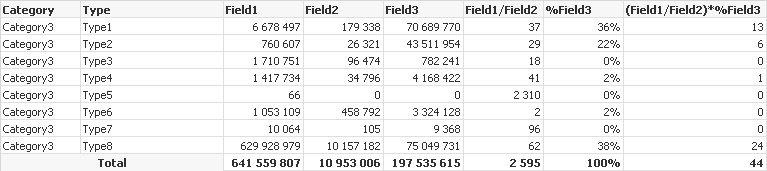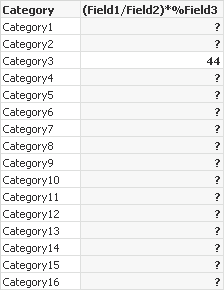# New to Qlik Sense

Discussion board where members can get started with Qlik Sense.

Announcements
Make your voice heard! Participate in the 2020 Wisdom of Crowds® Survey. BEGIN SURVEYPartner

## Help with Table Formula QlikSense?

Hi!

I really need some help with these formula, i believed it could be solved with aggregations but I haven´t reached the good one:

1.- The example data is grouped by Category and Type

2.- These are my Calculations in Excel for 1 category:3.- The Required Table is like this one:Tags (5)
1 Solution

Accepted Solutions
Highlighted
Valued Contributor

## Re: Help with Table Formula QlikSense?

sum(aggr((Field1/Field2)*(sum(aggr(Field3/sum(total<Category> Field3),Type,Category))),Type,Category))

2 Replies
Highlighted
Valued Contributor

## Re: Help with Table Formula QlikSense?

sum(aggr((Field1/Field2)*(sum(aggr(Field3/sum(total<Category> Field3),Type,Category))),Type,Category))

HighlightedPartner

## Re: Help with Table Formula QlikSense?

Thank You Very Much

This one was the correct one but it was very helpful

sum(Aggr((Sum(Field1)/Sum(Field2))*(sum(Aggr(sum(Field3)/sum(TOTAL <Category> Field3),Type,Category))),Type,Category))